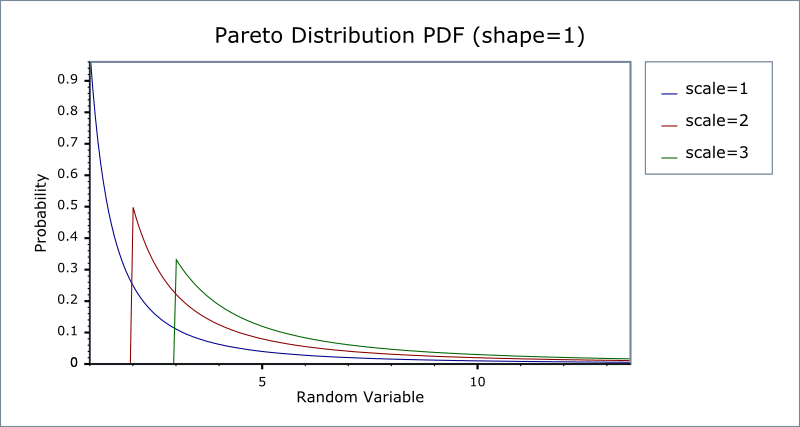#Boost C++ Libraries

...one of the most highly regarded and expertly designed C++ library projects in the world.

#### Pareto Distribution

`#include <boost/math/distributions/pareto.hpp>`
```namespace boost{ namespace math{

template <class RealType = double,
class Policy   = policies::policy<> >
class pareto_distribution;

typedef pareto_distribution<> pareto;

template <class RealType, class Policy>
class pareto_distribution
{
public:
typedef RealType value_type;
// Constructor:
pareto_distribution(RealType scale = 1, RealType shape = 1)
// Accessors:
RealType scale()const;
RealType shape()const;
};

}} // namespaces
```

The Pareto distribution is a continuous distribution with the probability density function (pdf):

f(x; α, β) = αβα / xα+ 1

For shape parameter α   > 0, and scale parameter β   > 0. If x < β  , the pdf is zero.

The Pareto distribution often describes the larger compared to the smaller. A classic example is that 80% of the wealth is owned by 20% of the population.

The following graph illustrates how the PDF varies with the scale parameter β:And this graph illustrates how the PDF varies with the shape parameter α:##### Member Functions
```pareto_distribution(RealType scale = 1, RealType shape = 1);
```

Constructs a pareto distribution with shape shape and scale scale.

Requires that the shape and scale parameters are both greater than zero, otherwise calls domain_error.

```RealType scale()const;
```

Returns the scale parameter of this distribution.

```RealType shape()const;
```

Returns the shape parameter of this distribution.

##### Non-member Accessors

All the usual non-member accessor functions that are generic to all distributions are supported: Cumulative Distribution Function, Probability Density Function, Quantile, Hazard Function, Cumulative Hazard Function, mean, median, mode, variance, standard deviation, skewness, kurtosis, kurtosis_excess, range and support.

The supported domain of the random variable is [scale, ∞].

##### Accuracy

The Pareto distribution is implemented in terms of the standard library `exp` functions plus expm1 and so should have very small errors, usually only a few epsilon.

If probability is near to unity (or the complement of a probability near zero) see also why complements?.

##### Implementation

In the following table α   is the shape parameter of the distribution, and β   is its scale parameter, x is the random variate, p is the probability and its complement q = 1-p.

Function

Implementation Notes

pdf

Using the relation: pdf p = αβα/xα +1

cdf

Using the relation: cdf p = 1 - (β   / x)α

cdf complement

Using the relation: q = 1 - p = -(β   / x)α

quantile

Using the relation: x = β / (1 - p)1/α

quantile from the complement

Using the relation: x = β / (q)1/α

mean

αβ / (β - 1)

variance

βα2 / (β - 1)2 (β - 2)

mode

α

skewness

kurtosis

kurtosis excess

##### References
 Copyright © 2006-2010, 2012, 2013 Paul A. Bristow, Christopher Kormanyos, Hubert Holin, Bruno Lalande, John Maddock, Johan Råde, Gautam Sewani, Benjamin Sobotta, Thijs van den Berg, Daryle Walker and Xiaogang Zhang Distributed under the Boost Software License, Version 1.0. (See accompanying file LICENSE_1_0.txt or copy at http://www.boost.org/LICENSE_1_0.txt)